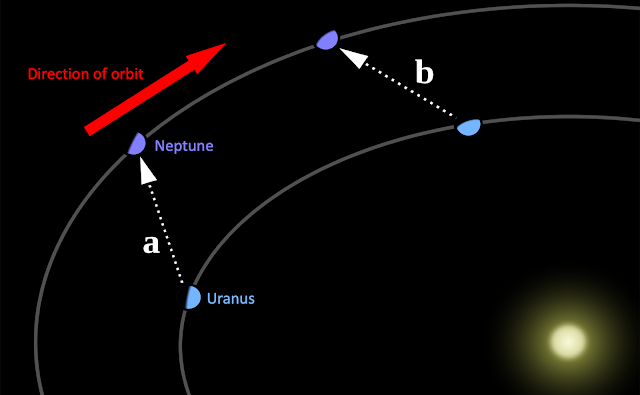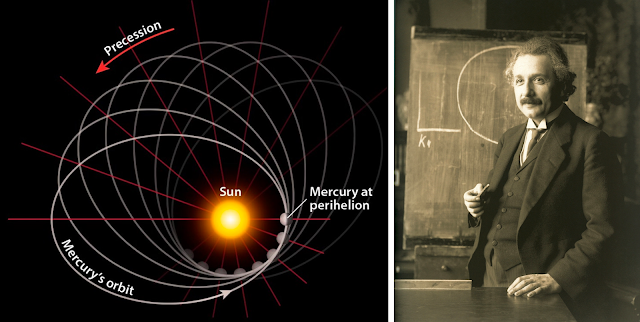### The Mystery of Mercury's Missing Arcseconds

After writing Astronomical Returns for over a year, I realized I'd never covered Mercury before!
Sorry little guy, this one's for you!
_______________________________________________________________

Last month, astronomers released an incredible decades-long observation of the star S2's rotating orbit around Sagittarius A*, the supermassive black hole at the center of our galaxy 25,000 light years away! The wayward star's peculiar path is analogous to what astronomers observed a century ago in Mercury's orbit around the Sun, further supporting Einstein's Theory of Relativity. But what does this petal-shaped pattern have to do with the fabric of spacetime?Newtonian physics would predict the static orange orbit, but S2's orbit very gradually rotates around the black hole due to relativistic effects

First, let's rewind the clock 400 years - in 1609, Johannes Kepler defined his laws of planetary motion, the first of which describes all orbits as ellipses (learn more here). Some 80 years later, Isaac Newton demonstrated that this elliptical path was directly the result of his law of universal gravitation, which stood untouched until the early 20th century. For a long time all was good and dandy; astronomers could use equations derived from Newton's work to accurately predict the stable elliptical orbits of say, the Moon around the Earth or the planets around the Sun. That is, until 1859 when French astronomer Urbain Le Verrier noticed inexplicable deviations in the orbit of Mercury after sifting through decades of old data - it appeared that the orbit of Mercury itself was gradually precessing (i.e. rotating) with each passing revolution!Similar to what astronomers recently saw in S2, Mercury's orbit displayed a wobble in its orbit around the Sun | Credit: NYT

Le Verrier and the rest of the astronomical community immediately set out to explain the cause of Mercury's precession, which was calculated to be occurring at a rate of 5600" / century, an incredibly slow yet still noticeable amount, even to 19th century astronomers (an arcsecond " is equal to 1/3600th of a degree, so Mercury's orbit rotates 1.56$^{\circ}$ every 100 years). Of this, Le Verrier was quickly able to attribute 5557" to gravitational perturbations from other bodies in the solar system (i.e. gravity from other planets also tugging on Mercury), explaining the majority of the precession. But that left 43 arcseconds unaccounted forUrbain Le Verrier and his "Theorie du Mouvement de Mercure"

So Le Verrier was forced to dig deeper for additional explanations - perhaps the Sun isn't a perfect sphere but more of an oblate spheroid, basically a sphere that's slightly squashed down so that it's wider than it is tall. While this is indeed the case, he found it barely contributed any additional arcseconds. Next, he surmised there might be an undiscovered planet he named "Vulcan" within the orbit of Mercury that was silently distorting its orbit. This may seem ridiculous to us now, but it wasn't an unreasonable theory at all - in fact, a few decades earlier, astronomers had predicted the presence of an 8th planet beyond the orbit of Uranus due to unusual motions in Uranus' orbit, and sure enough, Neptune was discovered in 1846 by... Urbain Le Verrier himself!! But the years dragged on, Le Verrier evenutally died in 1877, and still no trace of the missing Vulcan could be found. The 43 arcseconds remained unsolved, and as astronomers got more and more desperate, some even went so far as to guess that the inverse square law behind Newton's description of gravity was in fact wrong!At Position A, Neptune's gravity pulls Uranus forward in its orbit, whereas at Position B it hinders it | Source: Wikipedia

Enter Albert Einstein and his wacky theory about curved spacetime. Since Mercury is so close to the Sun, it's more heavily affected by its gravitational well, and General Relativity tells us that time slows down in a gravitational field. Additionally, since Mercury is the closest planet to the Sun, it has the highest orbital speed, and Special Relativity tells us that time slows down for objects that are moving fast (learn more here). Einstein predicted that the implications of relativity would finally crack the mystery of Mercury's orbit, thus providing the experimental proof he needed to convince the skeptics of his theory. Using calculations derived from Einstein's work, astronomers put forth the following expression to quantify how much relativity contributed to Mercury's orbital precession:

$\sigma = \frac{24 \thinspace \pi^3 \thinspace a^2}{T^2 \thinspace c^2 \thinspace (1 - e^2)}$

$\sigma =$ orbital precession, in radians / revolution (how much Mercury's orbit rotates each time it goes around the Sun)
$a$ = semi-major axis of Mercury's orbit (meters)
$T$ = orbital period (seconds)
$c$ = speed of light (meters / second)
$e$ = orbital eccentricity

** unfortunately the derivation of this expression is beyond my mathematical abilities, but if you're familiar with geodesics and the Einstein field equations, check this page out here

Plug in the numerical values into the equation, and we get:

$\sigma = \frac{24 \thinspace \pi^3 \thinspace (5.791 \cdot 10^{10})^2}{(87.969 \cdot 86400)^2 \thinspace (3 \cdot 10^8)^2 \thinspace (1 - 0.206^2)} = 5.01 \cdot 10^{-7}$ radians / revolution

And if you convert from radians / revolution to arcseconds / century, you get exactly 43" / century of orbital precession due to relativistic effects!

(1 radian = 57.3 degrees, 1 degree = 3600 arcseconds, 1 orbit of Mercury takes 87.969 days)Pretty darn amazing stuff, Mr. Einstein!!

Although Mercury's relativistic orbital precession is the most noticeable and the first to be calculated, with more precise measurements the same math can be done with all the other planets, and now with the latest announcement, even a star around a black hole!

Check out these two great videos here and here for more detail - they were extremely helpful to me as I researched this topic!